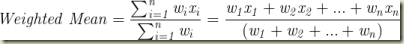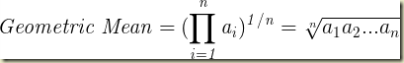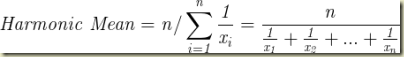# Measures of Central Tendency in Excel: Mean, Weighted Mean, Median, Mode, Geometric Mean, Harmonic Mean, and Weighted Harmonic Mean

## Measures of Central Tendency Overview

Measures of central tendency describe the center of a finite set of data or a theoretical distribution such as the normal distribution. Data is said to have a strong or weak central tendency based on measures of its dispersion such as standard deviation.

The following measures of central tendency can be calculated in Excel:

Mean (arithmetic mean) – the sum of all data values divided by the number of data values

Weighted mean – an arithmetic mean that incorporates weighting to certain data points

Median – the middle value that separates the upper half of the data set from the lower half of the data set

Mode – The most frequent value in the data set

Geometric mean – the nth root of the product of data, where there are n of these.

Harmonic mean – the reciprocal of the arithmetic mean of the reciprocals of the data values

Weighted harmonic mean

### Mean (Arithmetic mean) in Excel

The mean is simply the sum of a collection of data values in a data set divided by the number of data values in the data set. The mean is very sensitive to outliers, i.e., very high or low values. If significant outliers exist the median can be a more robust measure of central tendency than the mean. Many parametric statistical tests rely on the calculation of the mean. Parametric tests are statistical tests which require that the data set be distributed according to a specified distribution such as the normal distribution. Many nonparametric tests rely on the calculation of the median. Nonparametric tests do not require that the data set be distributed according to a specified distribution.

A population mean is denoted by µ, the Greek letter mu.

A sample mean is denoted by x_bar or x_avg.

The formula to calculate the mean is as follows:

The Excel formula to calculate the mean is the following:

AVERAGE(data range)

### Weighted Mean

The weighted arithmetic mean is used if certain individual data values should be given greater weight due to increased importance or due to a larger number of occurrences of specific values. The formula for the weighted mean is as follows:(Click On Image To See a Larger Version)

An instructor teaches two sections of the same course and gives the same test to both sections. The test average from section 1, which has 30 students, is 84. The test average of section 2, which has 20 students is 93. The average score of all students combined could be calculated using the weighted mean as follows:

Weighted Mean = (30*84 + 20*93) / (20 + 30) = 87.6

### Median in Excel

Half of the values of a data set are larger than the mean and half of the values are smaller than the mean. If there is no single value, then the median is defined as being the average of the two middle values. There is no widely-accepted symbol for the median so any symbol used to denote the median must be explicitly defined. The median is the 2nd quartile, 5th decile, and 50th percentile. The median and mode are the only measures of central tendency that can be applied to ordinal data. Ordinal data are data that are ranked but whose values have no specific numerical meaning.

The Excel formula to calculate the median is the following:

MEDIAN(data range)

### Mode in Excel

The mode is the most frequently occurring value in a data set. A data set is said to be unimodal if there is only one data value that occurs significantly more often than the other data values. The PDF (probability density function) of a unimodal data set has a global maximum and no other local maxima. The bell-shaped normal distribution is unimodal. A data set is said to be multimodal if there are more than one value that occurs significantly more often than the other values in a data set. The PDF of a multimodal data set has at least two local maxima. If the PDF has only two local maxima, the data set is said to be bimodal. Three local maxima in the PDF designate the data set as being trimodal.

The mode is one of the few descriptors that can be applied to a data set that is nominal. Nominal data are merely labels that have no numerical significance or ranking.

The shape of a data set’s distribution can be observed by creating a histogram of the data.

The Excel formula to calculate the mode is the following:

MODE(data range) – This function is compatible with Excel 2007 and earlier

MODE.SING(data range) – This function can be used in Excel 2010 or later. It returns the most frequently occurring value in a data set or data range.

MODE.MULT(data range) – This function can be used in Excel 2010 or later. It returns a vertical array of the most frequently occurring values in a data set or data range. For a horizontal array, use the following:

TRANSPOSE(MODE.MULT(data range))

Perhaps the quickest way to determine the modal values is to create an Excel histogram of the data. An example of this is shown as follows:

The histogram chart generated in Excel quickly shows that the data is bimodal and the two modes existing at 3 and 8.

### Geometric Mean in Excel

The geometric mean is the nth root of the product of n numbers.(Click On Image To See a Larger Version)

The Excel Formula for nth root is POWER(NUMBER,1/n). The geometric mean formula can be generalized in Excel as follows:

Geometric Mean =POWER(PRODUCT(data range),1/(COUNTA(data range))

COUNTA(data range) counts the number of cells in a range that are not empty.

A more direct way to calculate the geometric mean would be to use the following formula:

Geometric Mean = GEOMEAN(data range)

#### Combining Differently-Scaled Metrics into a Single Metric With Geometric Mean

The geometric mean provides a method to combine separate measures created on different scales into a single measure that is representative of all of the measures combined. The geometric mean is a way of normalizing the all measurements so that so that no one measure has a disproportionately large effect on the calculation of the geometric mean.

For example, products within a certain category might be rated for quality on a scale of 1 to 10 and also be rated for ease-of-use on a scale of 1 to 100. The geometric mean creates a single measure that combines both of these scores without allowing either of the scores to have a disproportionately large effect.

Brand A Quality Rating: 6

Brand A Ease-of-Use Rating: 70

Brand A Geometric mean = GEOMEAN(6,70) = 20.5

Brand B Quality Rating: 8

Brand B Ease-of-Use Rating: 25

Brand B Geometric mean = GEOMEAN(8,25) = 14.1

If the criteria of Quality Rating and Ease-of-Use Rating are considered equally important and are the two main criteria for selecting among brands within the category, Brand A would be the preferred brand as determined by its higher geometric mean of those two measures.

One very important characteristic of the geometric mean is the normalization of the scales of the different measures. The same percent change in either measure will produce the same change in the geometric mean.

Brand B Geometric mean = GEOMEAN(8,25) = 14.1

If Brand B’s Quality Rating is reduced by 50 percent from 8 to 4, the geometric mean now becomes the following:

Brand B Geometric mean = GEOMEAN(4,25) = 10

If Brand B’s Ease-of-Use Rating is reduced by 50 percent from 25 to 12.5, the geometric mean will also equal 10 as follows:

Brand B Geometric mean = GEOMEAN(8,12.5) = 10

#### Calculating Combined Growth Rate With Geometric Mean

Another important use of the geometric mean is to calculate a single “average” growth rate over periods of different growth rates that would produce the same overall amount of growth. For example, an investment of \$1,000 would grow to \$1,615 if it grew by 15 percent in the 1st year, 30 percent in the 2nd year, and 8 percent in the 3rd year. This can be calculated by the following:

1st year balance = \$1,000 * (1.15) = \$1,150

2nd year balance = \$1,150 * (1.30) = \$1,495

3rd year balance = \$1,495 * (1.08) = \$1,615

An average growth rate for all three periods would be calculated using the geometric mean as follows:

GEOMEAN(1.15,1.30,1.08) = 1.173154

The end balance after 3 years at this constant annual growth rate would be calculated as follows:

\$1,000 * (1.173154)3 = \$1,615

### Harmonic Mean in Excel

The harmonic mean is often used to calculate an average of different rates or ratios. The harmonic mean is also used to calculate an average of a set of numbers that might have significant outliers. The harmonic mean would provide a truer representation of the average than the arithmetic mean because outliers have a disproportionate effect on the arithmetic mean.

The harmonic mean is calculated by the following formula:(Click On Image To See a Larger Version)

The Excel formula to calculate the harmonic mean is the following:

Harmonic Mean = HARMEAN(data range)

#### Calculating the Average Rate Using the Harmonic Mean

One pump operates at 5 gallon/minute, a second pump operates at 7 gallons/minute, and a third pump operates at 9 gallons/minute. The average rate for all three pumps if each pump is used sequentially to pump the same amount as the other two pumps is calculated as follows:

HARMEAN(5,7,9) = 6.608392

If each pump was operated separately to pump out 40 gallons of water and they operated sequentially (one turned on immediately after another finished pumping 40 gallons of water), the average pumping volume over the entire time that it took all three pumps to collectively pump 120 gallons is 6.608392 gallons/min.

That can be verified as follows:

Total time = 120 gallons / (6.608392 gallons/minute) = 18.16 minutes

Total time = 40 gallons / (5 gal/min) + 40 gallons/(7 gal/min) + 40 gallons(9 gal/min) =

= 8 minutes + 5.7143 minutes + 4.4444 minutes = 18.16 minutes

#### Calculating the Average Speed Using the Harmonic Mean

If equal distances were individually travelled at different speeds, the harmonic mean would be used to calculate the average speed for the entire trip. For example Point A is 120 km from Point B. A train goes from Point A to Point B at 40 km/hour and then back to Point A at 60 km/hour, the average speed would be calculated using the harmonic mean as follows:

Average speed = HARMEAN(40,60) = 48 km/hour

This is verified as follows:

The total trip from A to B and then back to A would take the following amount of time travelling at 48 km/hour:

Total time = (120 km + 120 km)/(48 km/hour) = 5 hours

The total trip going from A to B at 40 km/hour and then from B to A at 60 km/hour would take the following amount of time:

Total time = (120 km)/(40 km/hour) + (120 km)/(60 km/hour) =3 hours + 2 hours = 5 hours

#### Calculating an Average when Significant Outliers Exist By Using the Harmonic Mean

One of the advantages that the harmonic mean has over the arithmetic mean is that outliers in the data set do not unduly skew the harmonic mean to the same degree that occurs with the arithmetic mean.

The following data set has a single significant outlier:

(3, 4, 6, 5, 7, 85, 6, 3, 5)

The harmonic mean of this data set is calculated in Excel as follows:

Harmonic mean = HARMEAN(3,4,6,5,7,85,6,3,5) = 4.988

The arithmetic mean of this data set is calculated in Excel as follows:

Arithmetic mean = AVERAGE(3,4,6,5,7,85,6,3,5) = 13.778

If the single outlier were removed, the harmonic and arithmetic means would be calculated as follows:

Harmonic mean = HARMEAN(3,4,6,5,7,6,3,5) = 4.462

Arithmetic mean = AVERAGE(3,4,6,5,7,6,3,5) = 4.875

#### The Harmonic Mean is the Preferred Method in Finance For Averaging Multiples

The harmonic mean gives equal weight to each data point and removes excessive influence that large outliers have on the arithmetic mean. Because of this quality, the harmonic mean is the preferred method in finance for averaging multiples such as the price/earnings ratio.

#### Calculating the Combined Resistance of Parallel Resistors Using the Harmonic Mean

The cumulative resistance of parallel resistor is calculated using the harmonic mean as follows:

Resistor 1 = 40Ω

Resistor 1 = 60Ω

Their combined resistance is place parallel to each other is the following:

Combined resistance = HARMEAN(40,60) = 48Ω

The harmonic mean is also in the same fashion to calculate the combined capacitance of capacitors arranged in series.

### Weighted Harmonic Mean in Excel

The harmonic mean formula above assumes each rate has produced the same output, i.e., the same distance was travelled at each of the different rates or the same amount of liquid was pumped at each of the different rates. The different output occur at different rates, a weighted harmonic mean should be applied. The formula for this is as follows:

There is no easy way of automating this formula in Excel. The weighting and data values must be individually entered into the equation as in the following example:

A person travels in a train at 40 km/hour for 100 km and at 60 km/hour for 150 km. Calculate the average speed for the entire trip.

The weighting for the first part of the trip, w1, is set to 2. The weighting for the second part of the trip, w2, is set to 3. Any set of number could be used for w1 and w2 as long as w1 / w2 = 2/3.

The weighted harmonic mean would then be calculated as follows:

Weighted Harmonic Mean = (2 + 3) / (2/40 + 3/60) = 50

The average speed for the entire trip is 50 km/hour. This can be verified as follows:

The total distance travelled was 250 km. At 50 km/hour, the trip would take 5 hours.

Traveling at 40 km/hour for 100 km would take 2.5 hours (100km / 40 km/hour = 2.5 hours)

Traveling at 60 km/hour for 150 km would take 2.5 hours (150km / 60 km/hour = 2.5 hours)

The total trip time is 5 hours.

Excel Master Series Blog Directory

Statistical Topics And Articles In

This Blog

You Will Become an Excel Statistical Master!

1.You mentioned in your blog about #PowerQualityaAnalysis is very nice to gain more knowledge about Harmonic Analysis. Harmonic distortions are one of the most common and irritating problems in industrial environment. We need to identify the source of harmonics and suppress them for a quality supply of power.We need to measure these harmonics at various points in the system and perform proper scientific analysis as per international standards.We need to measure these harmonics at various points in the system and perform proper scientific analysis as per international standards. If you have any queries about Harmonic Analysis, Please visit
Harmonic Analysis

2.Thanks for sharing your wealthy information. This is one of the excellent posts which I have seen. I go through your all of your blog, but this blog is the best one. It is really what I wanted to see hope in future you will continue for sharing such an excellent post.We appreciate that please keep going to write more content. We are the essay helper, we provide services all over the globe.Many times students are tensed for their projects and assignments, so at that time they can take online assignment help to complete it on time. There are experts available online who prepare the best essay writing service for you.

3.4.The ultimate goal of descriptive essay help services is to provide Descriptive Essay Writing Services and descriptive essay services since descriptive essay writing help seekers lack time to complete their custom descriptive essay writing services.

5.IEEE Final Year projects Project Centers in India are consistently sought after. Final Year Students Projects take a shot at them to improve their aptitudes, while specialists like the enjoyment in interfering with innovation. A IEEE Domain project Final Year Projects for CSE system development life cycle is essentially a phased project model that defines the organizational constraints of a large-scale systems project.

IT Company Employess Productivity usually increases when a company implements corporate training courses on latest technologies.
corporate training in chennai
It Companies need of Corporate training programme arises due to improvement in technology, need for getting better performance or as part of professional development. corporate training companies in chennai Corporate Training refers to a system of professional development activities provided to educate employees.
corporate training companies in india